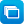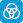# Mathematics / Year 10 / Statistics and Probability / Data representation and interpretation

Curriculum content descriptions

Construct and interpret box plots and use them to compare data sets (ACMSP249)

Elaborations
• understanding that box plots are an efficient and common way of representing and summarising data and can facilitate comparisons between data sets
• using parallel box plots to compare data about the age distribution of Aboriginal and Torres Strait Islander people with that of the Australian population as a whole
General capabilities
• Literacy Literacy
• Numeracy Numeracy
ScOT terms

Data analysis,  Box plots

## Refine results by

Year level 9-10
Resource type
Learning area Mathematics

## Refine by topic

Related topic### Graph investigator

Explore different graph types such as scatter plots, line graphs and histograms. Choose questions to investigate. For example, do girls have faster reaction times than boys? Select the appropriate data set. Then choose a useful graph type. Examine the data presented in the chosen graph type and draw a conclusion about the ...### Graph investigator: types of graphs

This learning object shows a variety of graphs used in data handling and explains their features, their different uses and their advantages. Graph types include stem-and-leaf plots, histogram, box-and-whisker, scatter plot, pie graph, line graph and column graph. This learning object is one in a series of seven objects. ...### Top drawer teachers

This is a website that covers five mathematical topics: fractions, mental computation, patterns, statistical literacy and ‘reasoning’. It also explores good teaching practice linked to classroom activities including how to address common misunderstandings. There is also a section for assessment, as well as worksheets with ...### Matchbox machine

Check whether a machine is packing most matchboxes with an acceptable number of matches (40–60 matches per box). Take a sample of 100 matchboxes and make a boxplot to analyse the results. Sort the sample data into four equal groups. Identify the range, median, first and third quartile values. Position the data points to ...### Using box plots to compare datasets

This is a collection of 15 digital curriculum resources that focuses on representing single-variable data, in the context of sampling and quality control in manufacturing, in box plots. Back-to-back (parallel) box and whisker plots are constructed and used to compare datasets. In both contexts, the significance of quartiles ...### Graph investigator: homework hours

Does the average number of hours spent on homework increase with year level? Select the appropriate data sets and choose a useful graph type to investigate this question. See the data presented in the chosen graph type and draw a conclusion about this question. Explore different graph types such as scatter plots, line graphs ...### Rice crisp machine

Check whether a machine is packing most bags with an acceptable number of rice crisps (20–35 rice crisps per packet). Take a sample of 100 packets and make a boxplot to analyse the results. Sort the sample data into four equal groups. Identify the range, median, first and third quartile values. Position the data points ...### Graph investigator: concentration [ESL]

Do students with better concentration have faster reaction times? Select the appropriate data sets and choose a useful graph type to investigate this question. See the data presented in the chosen graph type and draw a conclusion about this question. Explore different graph types such as scatter plots, line graphs and histograms. ...### Rice crisp machine: take a sample

Check whether a machine is packing most bags with an acceptable number of rice crisps (20–35 rice crisps per packet). Take a sample of 100 packets and make a boxplot to analyse the results. Sort the sample data into four equal groups. Identify the range, median, first and third quartile values. Position the data points ...### Graph investigator: hand preference

Do left-handed and right-handed students have different reaction times? Select the appropriate data sets and choose a useful graph type to investigate this question. See the data presented in the chosen graph type and draw a conclusion about this question. Explore different graph types such as scatter plots, line graphs ...### Fix the matchbox machine: speed

Check whether a machine is packing most matchboxes with an acceptable number of matches (40–60 matches per box). Look at boxplots made after taking samples of 100 matchboxes. Analyse the data and identify whether results are within the tolerance range. Adjust the machine's scoop size (if needed) to make it work correctly. ...### Fix the matchbox machine: scoop size

Check whether a machine is packing most matchboxes with an acceptable number of matches (40–60 matches per box). Look at boxplots made after taking samples of 100 matchboxes. Analyse the data and identify whether results are within the tolerance range. Adjust the machine's scoop size (if needed) to make it work correctly. ...### Matchbox machine: take a sample

Check whether a machine is packing most matchboxes with an acceptable number of matches (40–60 matches per box). Take a sample of 100 matchboxes and make a boxplot to analyse the results. Sort the sample data into four equal groups. Identify the range, median, first and third quartile values. Position the data points to ...### Graph investigator: hand preference [ESL]

Do left-handed and right-handed students have different reaction times? Select the appropriate data sets and choose a useful graph type to investigate this question. See the data presented in the chosen graph type and draw a conclusion about this question. Explore different graph types such as scatter plots, line graphs ...### Matchbox machine: plot the variation

Check whether a machine is packing most matchboxes with an acceptable number of matches (40–60 matches per box). Take a sample of 100 matchboxes and make a boxplot to analyse the results. Sort the sample data into four equal groups. Identify the range, median, first and third quartile values. Position the data points to ...### Fix the matchbox machine: scoop size and speed

Check whether a machine is packing most matchboxes with an acceptable number of matches (40–60 matches per box). Look at boxplots made after taking samples of 100 matchboxes. Analyse the data and identify whether results are within the tolerance range. Adjust the machine's scoop size or speed (if needed) to make it work ...### Graph investigator: concentration

Do students with better concentration have faster reaction times? Select the appropriate data sets and choose a useful graph type to investigate this question. See the data presented in the chosen graph type and draw a conclusion about this question. Explore different graph types such as scatter plots, line graphs and histograms. ...### Rice crisp machine: plot the variation

Check whether a machine is packing most bags with an acceptable number of rice crisps (20–35 rice crisps per packet). Take a sample of 100 packets and make a boxplot to analyse the results. Sort the sample data into four equal groups. Identify the range, median, first and third quartile values. Position the data points ...### Gapminder world

This is an interactive resource featuring an animated scatter plot displaying the development of over 200 countries between 1800 and 2011 based on data for two variables, the default being income per person and life expectancy, with population size represented by the size of the plotted points (bubbles). The graph has a ...### The First Fleet - dataset

The dataset provides information about 780 of the convicts transported to Australia on the First Fleet ships 'Alexander', 'Charlotte', 'Lady Penrhyn', 'Friendship', 'Prince of Wales' and 'Scarborough' in 1788. The dataset includes information on items such as the convict's name, occupation, crime, date of trial and term ...### Australian Historical Population Statistics to 2014 - dataset

The dataset provides statistics about the population of Australia in terms of age and sex from 1901 to 2014. It is made available by the Australian Bureau of Statistics (ABS). The dataset is in MS Excel format.### Scatter plots

Find out what a scatter plot is and how it can be used to investigate relationships between two variables. Analyse data for a pair of variables such as hand span and foot length. Enter your personal data and plot it with other data on a graph. Use a line of best fit to identify if there is a positive or negative relationship ...### Scatter plots: height and bellybutton height

Find out what a scatter plot is and how it can be used to investigate relationships between two variables. Analyse data for a pair of variables: a person's height and the height of their bellybutton measured from the floor. Enter your personal data and plot it with other data on a graph. Use a line of best fit to identify ...### Scatter plots: foot length and hand span

Find out what a scatter plot is and how it can be used to investigate relationships between two variables. Analyse data for a pair of variables: hand span and foot length. Enter your personal data and plot it with other data on a graph. Use a line of best fit to identify if there is a positive or negative relationship between ...### Scatter plots: create your own data

Find out what a scatter plot is and how it can be used to investigate relationships between two variables. Gather and analyse data for a pair of variables such as hand span and foot length. Enter your data set and plot it on a graph. Use a line of best fit to identify if there is a positive or negative relationship between ...### Scatter plots: age and reaction time

Find out what a scatter plot is and how it can be used to investigate relationships between two variables. Analyse data for a pair of variables: a person's age and the time it takes them to react to a signal. Enter your personal data and plot it with other data on a graph. Use a line of best fit to identify if there is ...### Graphing: line of best fit

Plot some data points on a graph. Estimate the position for a line of best fit. Use a graphing tool to check your estimate. Add more points to see how they affect the position of the line of best fit.### Scatter plots: about scatter plots

Find out what a scatter plot is and how it can be used to investigate relationships between two variables. Examine data for a pair of variables such as a person's height at the age of 2 and their height at the age of 22. Look at the data plotted on a graph. Notice how a line of best fit is used to identify if there is a ...